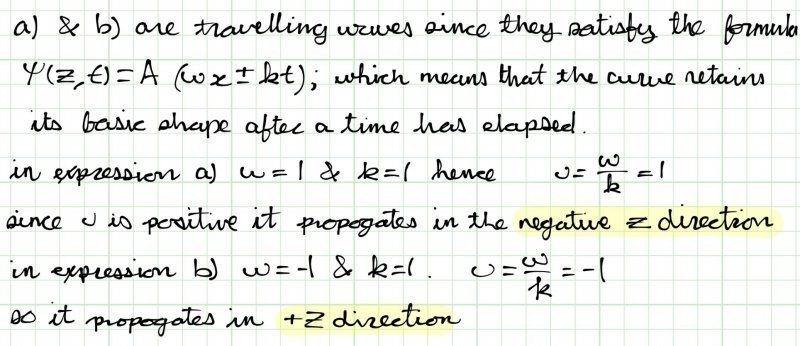# Are these travelling waves?

• shanepitts

## Homework Statement

Consider the following expressions:
(a) y(z,t)=A{sin[4π(t+z)]}^2
(b) y(z,t)=A(z-t)
(c) y(z,t)=A/[bz^(2)-t]

Which of them describe traveling waves? Prove it. Moreover, for the expressions that represent waves find the magnitude and direction of wave velocity.

y(z,t)=A(kx-wt)

v=w/k

## The Attempt at a SolutionLast edited:
Did you want to know something specific or are you just inviting comment? It is important to say.

I think you have worked harder than you needed to.
Definition: A shape of form ##y=f(z)## traveling in the +z direction with speed ##v## has form ##y(z,t)=f(z-vt)##
... this will be a traveling wave if it also satisfies the wave equation. (Do all such functions satisfy the wave equation?)

... a definition like that allows positive values of v to mean that the waveform propagates in the positive z direction - making it easier to keep track of minus signs.

For (a): ##y(z,t)=A\sin^2 4\pi(t+z)## ... this is a traveling wave with form ##f(z)=A\sin^2 4\pi z##
This means that ##z-vt = t+z \implies v=1\text{ (unit)} ## ... i.e. the wave propagates in the +z direction.
See how that somes easily?

There is also no need to go into wave numbers and angular frequencies.
You don't need the ##\pm## sign in your definitions unless you insist that the constants ##\omega## and ##k## can only take positive values.

Fortunately you don't have to prove that (c) is not a traveling wave.

•shanepitts